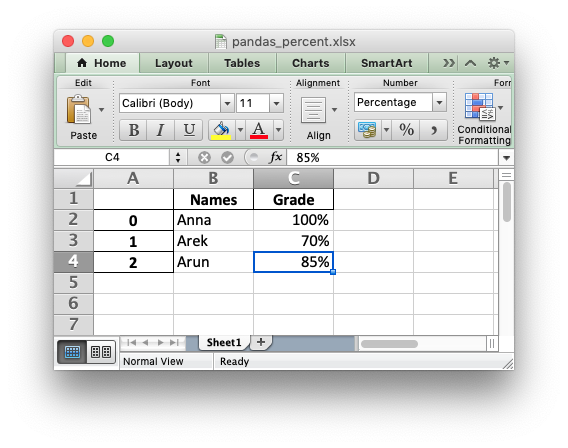# Example: Pandas Excel output with percentage formatting#

To create a percentage in Excel the data must be a number, must be divided by 100 and must have a percentage number format applied.

Here is a simple example of converting some string percentage data in a Pandas dataframe to percentage numbers in an xlsx file using XlsxWriter as the Pandas excel engine:```##############################################################################
#
# An example of converting some string percentage data in a Pandas dataframe
# to percentage numbers in an xlsx file with using XlsxWriter.
#
# Copyright 2013-2023, John McNamara, jmcnamara@cpan.org
#

import pandas as pd

# Create a Pandas dataframe from some data.
df = pd.DataFrame({"Names": ["Anna", "Arek", "Arun"], "Grade": ["100%", "70%", "85%"]})

# Convert the percentage strings to percentage numbers.

# Create a Pandas Excel writer using XlsxWriter as the engine.
writer = pd.ExcelWriter("pandas_percent.xlsx", engine="xlsxwriter")

# Convert the dataframe to an XlsxWriter Excel object.
df.to_excel(writer, sheet_name="Sheet1")

# Get the xlsxwriter workbook and worksheet objects.
workbook = writer.book
worksheet = writer.sheets["Sheet1"]

# Add a percent number format.